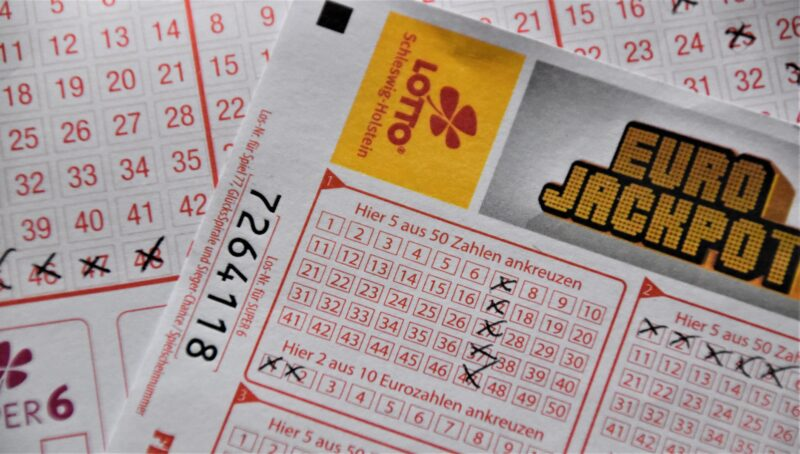# Python 威力彩進階分析 — 讀者提供想法## 第一階段資料爬取處理

import requests
import pandas as pd
from io import StringIO
import datetime as dt
url='https://www.pilio.idv.tw/lto/listbbk.asp?indexpage=1&orderby=new'
r=requests.get(url)
r.encoding='big5'
df=dfs
df.head()
url='https://www.pilio.idv.tw/lto/listbbk.asp?indexpage={}&orderby=new'
for i in range(2,55):
r=requests.get(url.format(i))
r.encoding='big5'

dfs=dfs

df=pd.concat([df,dfs])
df
df=df[df!='期數']
df.columns=['期數','日期','第一區','第二區','備註']
df=df[::-1]
df=df.set_index('期數',drop=True)
df['日期']=pd.to_datetime(df['日期'])
df['星期']=df['日期'].dt.dayofweek
df['星期']=df['星期']+1
df['第一區下一期']=df['第一區'].shift(-1)
df.head()
df_1=df['第一區'].str.split(',',expand=True)
df_1.columns=['第一','第二','第三','第四','第五','第六']
df_1['第二區']=df['第二區']
df_1['星期']=df['星期']
df_1=df_1.astype(int)
df_1['日期']=df['日期']
df_1.head()
df_1['第一區']=df_1['第一'].astype(str) + ',' + df_1['第二'].astype(str)+',' + df_1['第三'].astype(str)
df_1['第一區']=df_1['第一區']+ ',' + df_1['第四'].astype(str)+',' + df_1['第五'].astype(str)+ ',' + df_1['第六'].astype(str)
df_1.head()

## 第二階段進行資料分析

### 將下一期資料往前移

df_1['第一區下一期']=df_1['第一區'].shift(-1)
df_1['第二區下一期']=df_1['第二區'].shift(-1)
df_1.tail()

### 刪除遺漏值

df_1=df_1.iloc[:-1,:]
df_1['第二區下一期']=df_1['第二區下一期'].astype(int)
df_1.tail()

## 第一區號球分析

### 當這一期出現38號球下一期會出現幾號球

num=
df_1['sigh']=df_1[df_1.iloc[:,:6].isin(num)==True].count(axis=1)
target=df_1[df_1['sigh']==1]

from itertools import combinations
from collections import Counter

count=Counter()
for row in target['第一區下一期']:
row_list=row.split(',')
count.update(Counter(combinations(row_list,1)))
for key,value in count.most_common(10):

print(key,value,value/len(target))### 當這一期出現38號與35號下一期會出現幾號球

num=[38,35]
df_1['sigh']=df_1[df_1.iloc[:,:6].isin(num)==True].count(axis=1)
target=df_1[df_1['sigh']==2]

from itertools import combinations
from collections import Counter

count=Counter()
for row in target['第一區下一期']:
row_list=row.split(',')
count.update(Counter(combinations(row_list,1)))
for key,value in count.most_common(10):

print(key,value,value/len(target))

num=[38,35,18]
df_1['sigh']=df_1[df_1.iloc[:,:6].isin(num)==True].count(axis=1)
target=df_1[df_1['sigh']==3]

from itertools import combinations
from collections import Counter

count=Counter()
for row in target['第一區下一期']:
row_list=row.split(',')
count.update(Counter(combinations(row_list,1)))
for key,value in count.most_common(10):

print(key,value,value/len(target))

## 第二區號球分析

### 當這一期第二區出現三號下一期第二區會出現幾號球

i=3
df_1[df_1['第二區']==i]['第二區下一期'].value_counts()/len(df_1[df_1['第二區']==i])

## 結論與建議Grandma